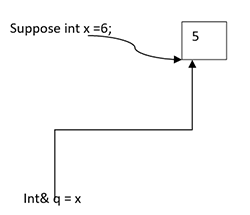# Reference in C++

by Yogesh Khanna 17-Sep-18

0 402

Reference is called an alias created for any variable.

suppose int x =6;

When we are making such declaration, a random memory location is selected in the stack and can be accessed by x and value will be stored as 6 and will have some address i.e. maybe 1000. Reference is known as creating another name for the existing variable

i.e. datatype & reference name =variable name;

int& q = x;Now you can access the value by another name i.e. q and if we try p++ the value of stack become 6

Pointed to be noted

No separate memory location is created when you create any reference unlike pointer as in pointers we need

int*p =&x;

In this case, one memory location is created for p, it will store the address of xi.e.1000 and it will have its own address i.e. 2000 and in reference, no memory location is created.

Following are the rules that are needed to know while referencing:

1) When you define reference, you need to initialize it with any variable
2) We can create an array of pointers but we can't able to create an array of references
3) Reference can never be initialized to null unlike pointers
4) Reference is fixed. Once you initialize it, resign of reference is not permitted, unlike pointers.

Important Application of reference in c++

Pass by reference is not available in our c programming language.

As in example

`int r=20;int q= 30;swap(r, q);void swap(int p,int s) // call by value example{int temp;temp=p;p=s;s=temp}`

In this, the value would not get swap by the pass by value technique. The reason behind is that when we make the call swap(r,q) the r and q are already there in stack with value 10 and 20 respectively. But when you pass by value void swap(int p,int s), the copies of that value is created in the form of p and s i.e 10 and 20 respectively. In this values are swapped instead of original integers. For that,we need to use pass by address.

`int r=20;int q= 30;swap(&r,& q);void swap(int* p,int *s){int temp;temp=*p;*p=*s; //call by address*s=temp}`

This value of address can be eliminated with reference as in following example

`main(){int r=20;int q=30;swap(r,q);void swap(int& p, int&q){int temp;iemp=p;p=q;q=temp}`

Thus in C++, we have 3 methods to pass parameters i.e.

1) pass by address
2) pass by reference
3) pass by value

Call by Reference in C++
Now we have already understood the function, function definition and how the function can be called. We have learned the examples of calling the function but they all are the example of the call by value now we will learn about the other method of calling function i.e. call by reference. The original values are modified by the call by reference because references or address are passed and both the actual and formal arguments share the same address space. Therefore value modified in the function affect the outside function also

Call by Reference with the help of program
// example of the call by reference in C++ program to swap two numbers

`#include <iostream>using namespace std;void swap(int& j, int& k){              int m = j;              j = k;              k = m;}int main(){              int c = 56, d = 76;              cout << "Before Swap of two numbers\n";              cout << "c = " << c << " d = " << d << "\n";              swap(c, d);              cout << "After Swapping with the pass by reference method\n";              cout << "c = " << c << " d = " << d << "\n";}`
Output:

Before swap of two numbers
c = 56  d = 76

After swapping with the pass by reference method
c = 76  d = 56

 CALL BY REFERENCE CALL BY VALUE Copy of addresses of value is passed to the function. In call by value, only values are passed through the function. Formal and actual parameters are constructed in the same memory location i.e. no separate memory location is created unlike pointers. Formal and actual parameters will be constructed in a different locations. Modifications inside the functions effect outside the function. Modifications inside the function does not affects outside function.

Share:

## Comments

No Comments Yet.